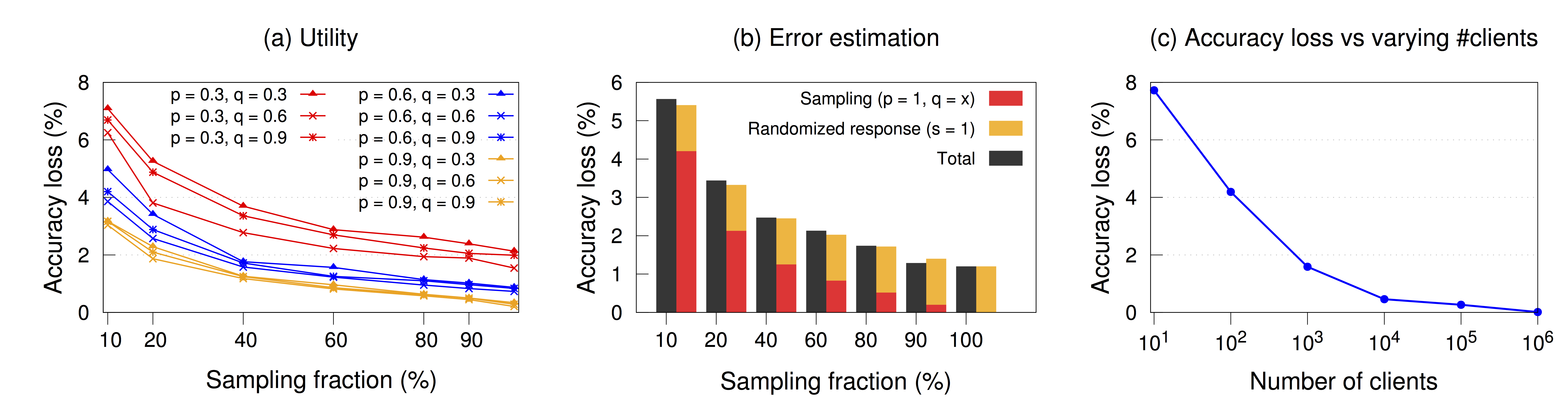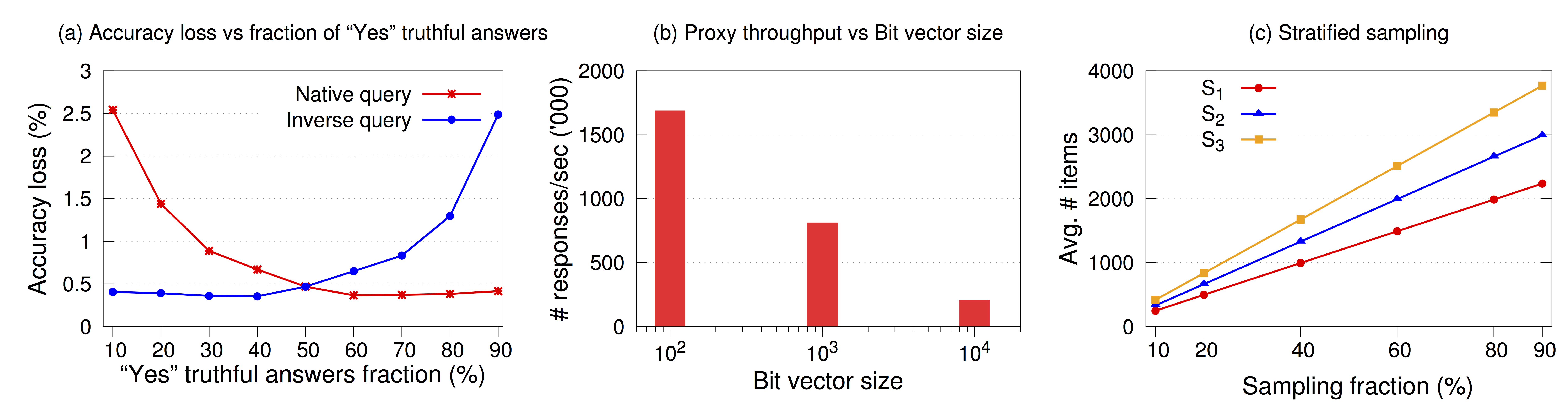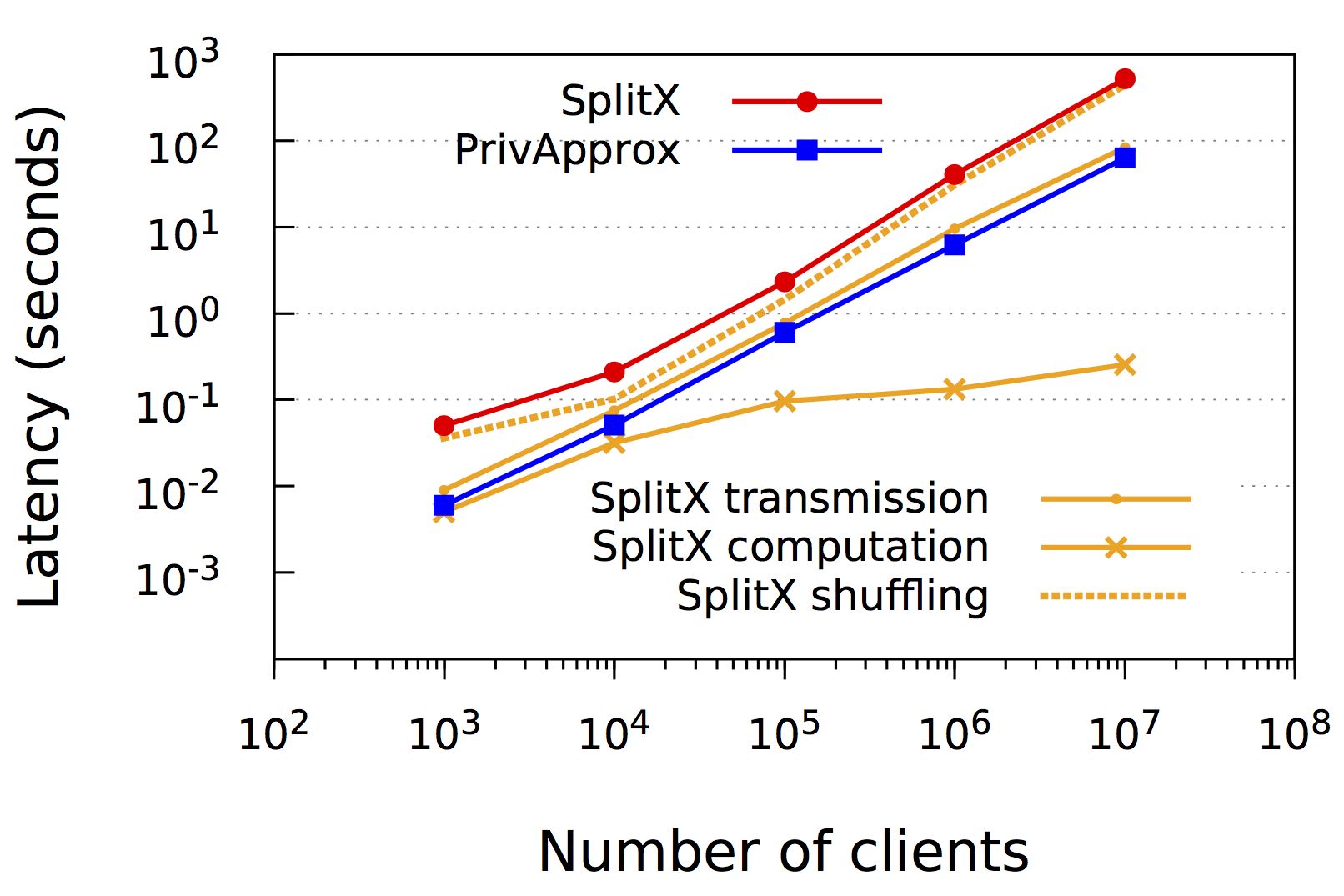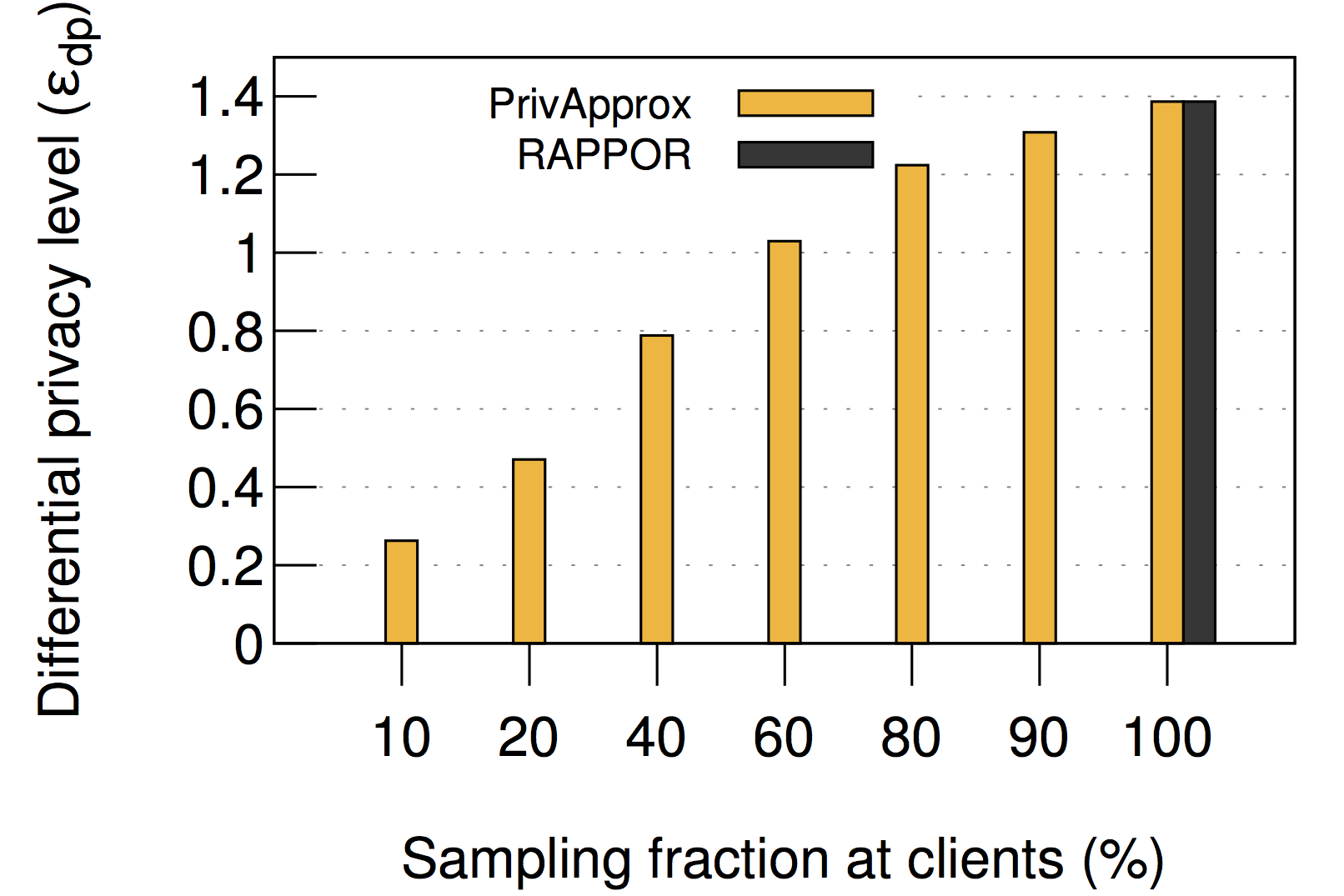# Micro-benchmarks Evaluation

We evaluate PrivApprox using a series of microbenchmarks. For all microbenchmark measurements, we report the average over $100$ runs.

## Results

### Effect of sampling and randomization parameters

We first measure the effect of randomization parameters on the utility and privacy guarantee of the query results. In particular, the utility is measured by the query result’s accuracy loss (Equation 3), and privacy is measured by the level of achieved zero-knowledge privacy (Theorem C.4. in the technical report). For the experiment, we generated $10,000$ original answers randomly, 60% of which are “Yes” answers. The sampling parameter $s$ is set to $0.6$.

Table 1 shows that different settings of the two randomization parameters, $p$ and $q$, do affect the utility and the privacy guarantee of the query results. The higher $p$ means the higher probability that a client responds with its truthful answer. As expected, this leads to higher utility (i.e., smaller accuracy loss $\eta$) but weaker privacy guarantee (i.e., higher privacy level $\epsilon$). In addition, Table 1 also shows that the closer we set the probability $q$ to the fraction of truthful “Yes” answers (i.e., $60%$ in this microbenchmark), the higher utility the query result provides. Nevertheless, to meet the utility and privacy requirements in various scenarios, we should carefully choose the appropriate $p$ and $q$. In practice, the selection of the $\epsilon$ value depends on real-world applications.

pqAccuracy loss ($\eta$)Privacy level ($\epsilon$)
0.30.30.02781.7047
0.30.60.02621.3862
0.30.90.02681.2527
0.60.30.01412.5649
0.60.60.01282.0476
0.60.90.01361.7917
0.90.30.10364.1820
0.90.60.00793.5263
0.90.90.01023.1570

Table 1: Utility and privacy of query results with different randomization parameters $p$ and $q$.

We also measured the effect of sampling parameter on the accuracy loss. Figure 1 (a) shows that the accuracy loss decreases with the increase in sampling fraction, regardless of the settings of randomization parameters $p$ and $q$. The benefits reach diminishing returns after $80%$ sampling fraction.Figure 1: (a) Accuracy loss with varying sampling and randomization parameters. (b) Error estimation during the randomized response process and sampling process, combined and individually. (c) Accuracy loss with varying # of clients.

### Error estimation

To analyze the accuracy loss, we first separately measured the accuracy loss caused by running, individually, the sampling process and the randomized response process. For the comparison, secondly, we also computed the total accuracy loss after running the two processes in succession as in PrivApprox. For the experiment, we set he number of original answers to $10,000$ with $60%$ of which being “Yes” answers. We compute the accuracy loss of the randomized response process separately by setting the sampling parameter to $100%$ ($s = 1$) and the randomized response parameters $p$ and $q$ to $0.3$ and $0.6$, respectively. Meanwhile, we measure the accuracy loss of the sampling process without the randomized response process by setting $p$ to $1$.

Figure 1 (b) represents that the accuracy loss during the two experiments is statistically independent to each other. In addition, the accuracy loss of the two processes can effectively be added together to calculate the total accuracy loss.

### Effect of the number of clients

We next analyzed how the number of participating clients affects the utility of the results. For the experiment, we fix the sampling and randomization parameters $s$, $p$ and $q$ to $0.9$, $0.9$ and $0.6$, respectively, and set the fraction of truthful “Yes” answers to $60%$.

Figure 1 (c) shows that the utility of query results improves with the increase in the number of participating clients, and few clients (e.g., $<100$) may lead to low-utility query results.

### Effect of the fraction of truthful answers

We also measured the utility of both the native and the inverse query results with different fractions of truthful “Yes” answers. For the experiment, we still keep the sampling and randomization parameters $s$, $p$ and $q$ to $0.9$, $0.9$ and $0.6$, respectively, and set the total number of answers to $10,000$.

Figure 2 (a) shows that PrivApprox achieves higher utility as the fraction of truthful “Yes” answers gets closer to $60$% (i.e., the $q$ value). In addition, when the fraction of “Yes” truthful answers $y$ is too small compared to the $q$ value (e.g., $y = 0.1$), the accuracy loss is ($2.54$%). However, by using the query inversion mechanism (see §3.3.3 in the technical report), we can significantly reduce the accuracy loss ($0.4$%).Figure 2: (a) Accuracy loss for the native and inverse query results with different fractions of truthful “Yes” answers. (b) Throughput of proxies with different bit-vector sizes for the query answer. (c) Average number of sampled data items after stratified sampling with different sampling fractions.

### Effect of answer’s bit-vector sizes

We also measured the throughput at proxies with various sizes for the bit-vector of client answers ($A[n]$ ). We conducted this experiment with a $3$-node cluster. Figure 2 (b) shows that the throughput, as expected, is inversely proportional to the answer’s bit-vector sizes.

### Effect of stratified sampling

To illustrate the use of stratified sampling, we generated a synthetic data stream with three different stream sources $S_1$, $S_2$, $S_3$. Each stream source is created with an independent Poisson distribution. In addition, the three stream sources have an arrival rate of $3:4:5$ data items per time unit, respectively. The computation window size is fixed to $10,000$ data items.

Figure 2 (c) shows the average number of selected items of each stream source with varying sample fractions using the stratified sampling mechanism. As expected, the average number of sampled data items from each stream source is proportional to its arrival rate and the sample fractions.

### Computational overhead of crypto operations

We also compared the computational overhead of crypto operations used in PrivApprox and prior systems. In particular, these crypto operations are XOR in PrivApprox, RSA, Goldwasser-Micali , and Paillier . For the experiment, we measured the number of crypto operations that can be executed on: (i) Android Galaxy mini III smartphone running Android 4.1.2 with a 1.5 GHz CPU; (ii) MacBook Air laptop with a 2.2 GHz Intel Core i7 CPU running OS X Yosemite 10.10.2; and (iii) Linux server running Linux 3.15.0 equipped with a 2.2 GHz CPU with 32 cores.

Table 2 shows that the XOR operation is extremely efficient compared with the other crypto mechanisms. This highlights the importance XOR encryption for our system design.

Encryption      Decryption
Phone Laptop Server Phone Laptop Server
RSA93716$\times$2,770341$\times$ 4,909275$\times$ 12625890$\times$69823666$\times$ 85926401$\times$
Goldwasser2,1067$\times$17,06455$\times$22,90259$\times$ 12725686$\times$6,3292610$\times$ 7,0683209$\times$
Paillier116129$\times$ 4891930$\times$ 5792335$\times$ 7245308$\times$ 25066076$\times$ 30973392$\times$
PRIVAPPROX15,026 943,902 1,351,937 3,262,186 16,519,076 22,678,285

Table 2: Comparison of crypto overheads (# operations/sec). The public-key crypto schemes use a 1024-bit key.

### Throughput at clients

We also measured throughput at clients. In particular, we measured the number of operations per second that can be executed at clients for the query answering process. For this experiment, we used the same set of devices as in the previous experiment.

Table 3 presents the throughput at clients. To further investigate the overheads, we measured the individual throughput of three sub-processes in the query answering process: (i) database read, (ii) randomized response, and (iii) XOR encryption. The result indicates that the performance bottleneck in the answering process is actually the database read operation.

No. of operations/secPhoneLaptopServer
Randomized response168,938418,6681,809,662
XOR encryption15,026943,9021,351,937
Total1,11617,23622,026

Table 3: Throughput (# operations/sec) at clients.

First, we compared PrivApprox with SplitX , a high-performance privacy-preserving analytics system. We compare the latency incurred at proxies in both PrivApprox and SplitX. SplitX is geared towards batch analytics, but can be adapted to enable privacy-preserving data analytics over data streams. We compare the latency incurred at proxies in both PrivApprox and SplitX.Figure 3: Latency comparison b/w SplitX and PrivApprox.

Figure 3 shows that, with different numbers of clients, the latency incurred at proxies in PrivApprox is always nearly one order of magnitude lower than that in SplitX. The reason is simple: unlike PrivApprox, SplitX requires synchronization among its proxies to process query answers in a privacy-preserving fashion. This synchronization creates a significant delay in processing query answers, making SplitX unsuitable for dealing with large-scale stream analytics. More specifically, in SplitX, the processing at proxies consists of a few sub-processes including adding noise to answers, answer transmission, answer intersection, and answer shuffling; whereas, in PrivApprox, the processing at proxies contains only the answer transmission. Figure 3 also shows that with $10^6$ clients, the latency at SplitX is $40.27$ sec, whereas PrivApprox achieves a latency of just $6.21$ sec, resulting in a $6.48\times$ speedup compared with SplitX.

Next, we compared PrivApprox with a recent privacy-preserving analytics system called RAPPOR. Similar to PrivApprox, RAPPOR applies a randomized response mechanism to achieve differential privacy. To make an “apple-to-apple” comparison between PrivApprox and RAPPOR in terms of privacy, we make a mapping between the system parameters of the two systems. We set the sampling parameter $s = 1$, and the randomized parameters $p = 1- f$, $q = 0.5$ in PrivApprox, where $f$ is the parameter used in the randomized response process of RAPPOR. In addition, we set the number of hash functions used in RAPPOR to $1$ ($h = 1$) for a fair comparison. In doing so, the two systems have the same randomized response process. However, since PrivApprox makes use of the sampling mechanism before performing the randomized response process, PrivApprox achieves stronger privacy. Figure 4 shows the differential privacy level of RAPPOR and PrivApprox with different sampling fractions $s$.Figure 4: Differential privacy level comparison b/w RAPPOR and PrivApprox.

It is worth mentioning that, by applying the sampling mechanism, PrivApprox achieves stronger privacy (i.e., zero-/knowledge privacy) for clients. The comparison between differential privacy and zero-knowledge privacy is presented in Theorem C.4. in the technical report.